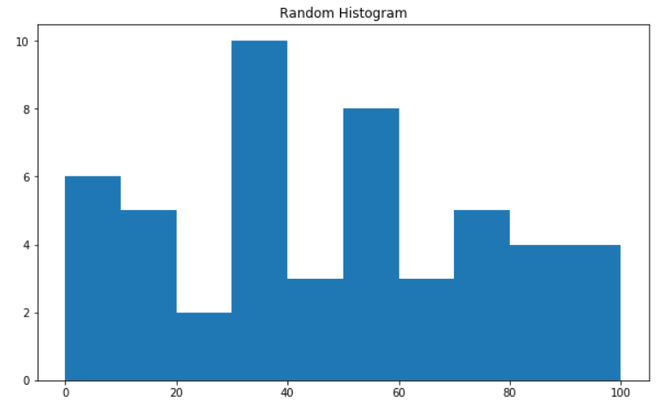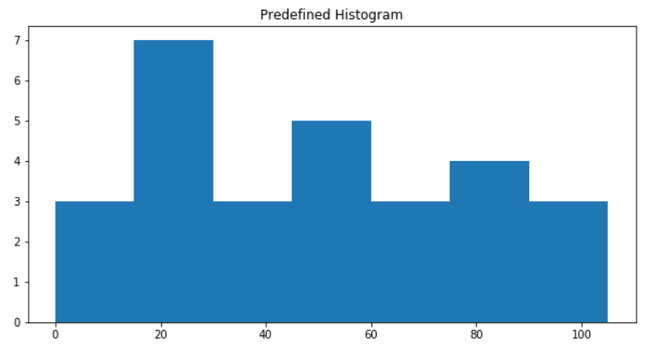Related Articles

# Compute the histogram of a set of data using NumPy in Python

• Last Updated : 05 Sep, 2020

Numpy provides us the feature to compute the Histogram for the given data set using NumPy.histogram() function. The formation of histogram depends on the data set, whether it is predefined or randomly generated.

Syntax : numpy.histogram(data, bins=10, range=None, normed=None, weights=None, density=None)

Case 1: Computing the Numpy Histogram with the help of Random Data set

## Python3

 `# import Numpy and matplotlib``from` `matplotlib ``import` `pyplot as plt``import` `numpy as np`` ` ` ` `# Creating random dataset``data_set ``=` `np.random.randint(``100``, size``=``(``50``))`` ` `# Creation of plot``fig ``=` `plt.figure(figsize``=``(``10``, ``6``))`` ` `# plotting the Histogram with certain intervals``plt.hist(data_set, bins``=``[``0``, ``10``, ``20``, ``30``, ``40``, ``50``,``                         ``60``, ``70``, ``80``, ``90``, ``100``])`` ` `# Giving title to Histogram``plt.title(``"Random Histogram"``)`` ` `# Displaying Histogram``plt.show()`

Output:In the above example, we created a random data set using np.random.randint() and plot the Numpy Histogram

Case 2: Computing the Numpy Histogram with the help of Pre-defined Data set

## Python3

 `# import Numpy and matplotlib``from` `matplotlib ``import` `pyplot as plt``import` `numpy as np`` ` ` ` `# Using predefined dataset``data_set ``=` `[``45``, ``85``, ``95``, ``10``, ``58``, ``77``, ``92``, ``72``, ``52``,``            ``22``, ``32``, ``5``, ``95``, ``2``, ``23``, ``24``, ``50``, ``40``, ``60``,``            ``69``, ``44``, ``80``, ``21``, ``15``, ``17``, ``55``, ``21``, ``88``]`` ` `# Creation of plot``fig ``=` `plt.figure(figsize``=``(``10``, ``5``))`` ` `# plotting the Histogram with certain intervals``plt.hist(data_set, bins``=``[``0``, ``15``, ``30``, ``45``, ``60``, ``75``, ``90``, ``105``])`` ` `# Giving title to Histogram``plt.title(``"Predefined Histogram"``)`` ` `# Displaying Histogram``plt.show()`In the above example, we take a predefined data set and plot the Numpy Histogram.

Attention geek! Strengthen your foundations with the Python Programming Foundation Course and learn the basics.

To begin with, your interview preparations Enhance your Data Structures concepts with the Python DS Course. And to begin with your Machine Learning Journey, join the Machine Learning – Basic Level Course

My Personal Notes arrow_drop_up